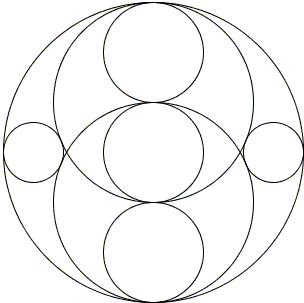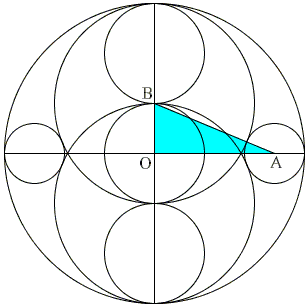## Sangaku with 8 Circles

This one sangaku appears to come with a solution. I can't read what it is about, but what is universally readable clearly points to the Pythagorean theorem.As out of eight circles six have obvious relations between their radii, the question is probably of finding the radius of the two small circles in terms of the radius of a bigger one.

SolutionAssume the radius of the biggest circle is 3r, then r is the radius of each circle in the vertical triplet, and 2r is the radius of the two bigger twins. Let x be the unknown radius of the small circles. In ΔABO,

 AB = 2r + x, OB = r, OA = 3r - x.

By the Pythagorean theorem

 r² + (3r - x)² = (2r + x)²

which leads to 6r² = 10rx, or x = 3r/5. If R = 3r is the radius of the big circle, then x = R/5.## Sangaku